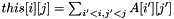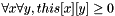util::PrefixSumArray2DOfTBracket< T > Class Template Reference

skeleton of prefix sum array using the bracket notation for 2d partitioning algorithm (this class does not actually exist). More...

#include <util/template.hpp>

Detailed Description

template<typename T> class util::PrefixSumArray2DOfTBracket< T >

skeleton of prefix sum array using the bracket notation for 2d partitioning algorithm (this class does not actually exist).

Any type that provides the following functions can be used safely with 2D partitioning algorithms that use the bracket notation (all of them right now). This class does not actually exist, it is provided for documentation purpose only. Notice that since the types are given as template parameter to the partitioning algorithm, there is no need to inherit from this class.

Intuitively, this class represents the prefix sum of an array (we call A) so that. Therefore, it is similar to the "Pascal-style"; the first column and the first row are composed exclusively of 0. and if A if of size XxY, the prefixsum array is indexed from 0 to X (included) in the first dimension and from 0 to Y (included) in the second dimension.

Types that respect this prototype include: util::Prefix2D, util::SubPSA, util::TransposePrefix2D.

One can use the function util::constraint_psa to verify the validity of a 2D prefixs sum array.

List of all members.

Public Member Functions

int prefixsizeX () const
returns the size of the first dimension of the prefix sum array.
int prefixsizeY () const
returns the size of the second dimension of the prefix sum array.
Something operator[] (int x) const

Member Function Documentation

template<typename T >
 Something util::PrefixSumArray2DOfTBracket< T >::operator[] ( int x ) const

Since [][] is not a C++ operator, operator[] must return an object whose type allow the operator[] to be applied. So that one can use this[x][y]. Something must define a function of prototype equivalent to "const T& operator[] (int y) const" that allows value of y between 0 and prefixsizeY()-1.

x must be between 0 and prefixsizeX()-1.

Since the represented load matrix is of positive (or null) integers, it must respect the constraintsand.

template<typename T >
 int util::PrefixSumArray2DOfTBracket< T >::prefixsizeX ( ) const

returns the size of the first dimension of the prefix sum array.

So, if this object represents the prefix sum of an array A of size XxY, this function should return X+1.

template<typename T >
 int util::PrefixSumArray2DOfTBracket< T >::prefixsizeY ( ) const

returns the size of the second dimension of the prefix sum array.

So, if this object represents the prefix sum of an array A of size XxY, this function should return Y+1.

The documentation for this class was generated from the following file: